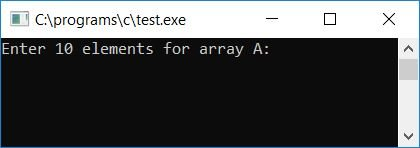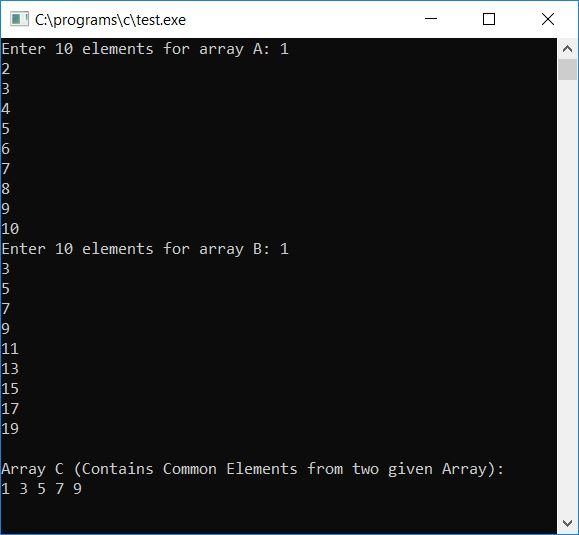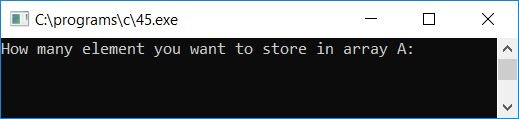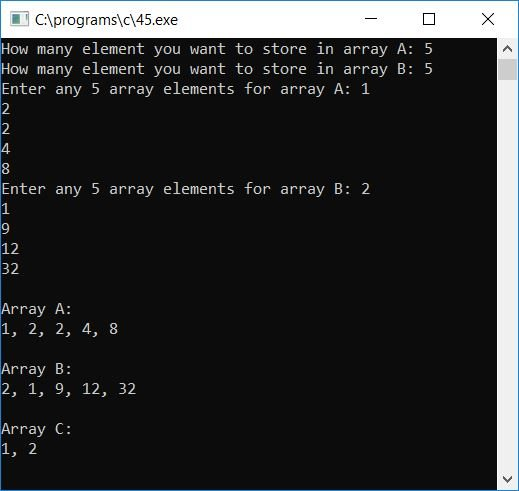# C Program to Find and Print Common Elements from Two Arrays

In this article, we will learn how to create a program that allows the user to enter any 10 or more array elements for two arrays and then put all the common elements from both arrays in a third array. In this case, we must place common elements in the third array while avoiding duplicates.Finally, print the value of the third array as output.

## Find Common Elements from Two Arrays in C

The question is: write a program in C that reads 10 elements from two arrays, say a and b, from the user and then puts common elements from both arrays in a third array without putting any duplicate elements, then prints the value of the third array. The program given below is the answer to this question:

```#include<stdio.h>
#include<conio.h>
int main()
{
int a, b, c, i, j, k=0, x, count;
printf("Enter 10 elements for array A: ");
for(i=0; i<10; i++)
scanf("%d", &a[i]);
printf("Enter 10 elements for array B: ");
for(i=0; i<10; i++)
scanf("%d", &b[i]);
for(i=0; i<10; i++)
{
for(j=0; j<10; j++)
{
if(a[i]==b[j])
{
count = 0;
for(x=0; x<k; x++)
{
if(a[i]==c[x])
count++;
}
if(count==0)
{
c[k] = a[i];
k++;
}
}
}
}
printf("\nArray C (Contains Common Elements from two given Array):\n");
for(i=0; i<k; i++)
printf("%d ", c[i]);
getch();
return 0;
}```

As the above program was written in the Code::Blocks IDE, here is the sample run that you will also get on your output screen after a successful build and run. Here is the first snapshot of the sample run:Now supply any 10 array elements for both the arrays and then press the ENTER key to see the common elements from both the arrays as output, as shown here in the second snapshot of the sample run:Here are some of the main steps used in the above program:

• Receive any 10 elements for the first array, say a.
• Again, receive any 10 elements for the second array, say b.
• Create a for loop that runs from 0 to 9.
• Inside this, create another for loop that also runs from 0 to 9.
• We have created two for loops here to compare every element of the first array to every element of the second array.
• The two for loop in this case is designed so that the 0th element of the first array is compared to all 10 elements of the second array, then the 1st element of the first array is compared to all 10 elements of the second array,... and the 9th (last) element of the first array is compared to all 10 elements of the second array.
• Inside the inner for loop, use an if statement to check whether the current element of both the arrays is equal to each other or not.
• If it is, then again check whether this element is already present inside the third array, say c[] or not.
• If it is, then increment the value of any variable, say count, to check later whether count holds its original value of 0 or not.
• If it holds its original value, then we have to put the current element inside the third array; otherwise, if count has some value, then it means that the current element already exists inside the third array.
• Ignoring the duplicate element, continue to check for the next match.
• After placing all matching elements from both arrays (without duplicate elements), exit both the for loops.
• At last, print the value of the third array that shows all the common elements from both the given arrays.

Let's modify the above program and allow the user to provide the size for both the array and its elements. Then put the common elements in the third array without any duplicate elements. Finally, print out all three arrays as output. Here is the program:

```#include<stdio.h>
#include<conio.h>
int main()
{
int a, b, c, i, limitA, limitB, j, k=0, x, count=0;
printf("How many element you want to store in array A: ");
scanf("%d", &limitA);
printf("How many element you want to store in array B: ");
scanf("%d", &limitB);
printf("Enter any %d array elements for array A: ", limitA);
for(i=0; i<limitA; i++)
scanf("%d", &a[i]);
printf("Enter any %d array elements for array B: ", limitB);
for(i=0; i<limitB; i++)
scanf("%d", &b[i]);
for(i=0; i<limitA; i++)
{
for(j=0; j<limitB; j++)
{
if(a[i]==b[j])
{
count=0;
for(x=0; x<k; x++)
{
if(a[i]==c[x])
count++;
}
if(count==0)
{
c[k]=a[i];
k++;
}
}
}
}
printf("\nArray A:\n");
for(i=0; i<limitA; i++)
{
if(i==(limitA-1))
printf("%d", a[i]);
else
printf("%d, ", a[i]);
}
printf("\n\nArray B:\n");
for(i=0; i<limitB; i++)
{
if(i==(limitB-1))
printf("%d", b[i]);
else
printf("%d, ", b[i]);
}
printf("\n\nArray C:\n");
for(i=0; i<k; i++)
{
if(i==(k-1))
printf("%d", c[i]);
else
printf("%d, ", c[i]);
}
getch();
return 0;
}```

The program was built and run in the Code::Blocks IDE. Here is the first snapshot of the sample run:Let's provide the size and elements for both the arrays. Press the ENTER key to see both the arrays along with a third array that contains the common elements from both the given arrays, as shown in the second snapshot of the sample run here:C Quiz

« Previous Program Next Program »